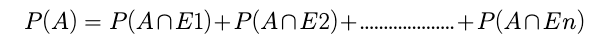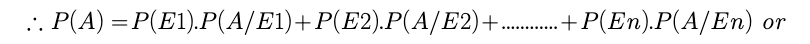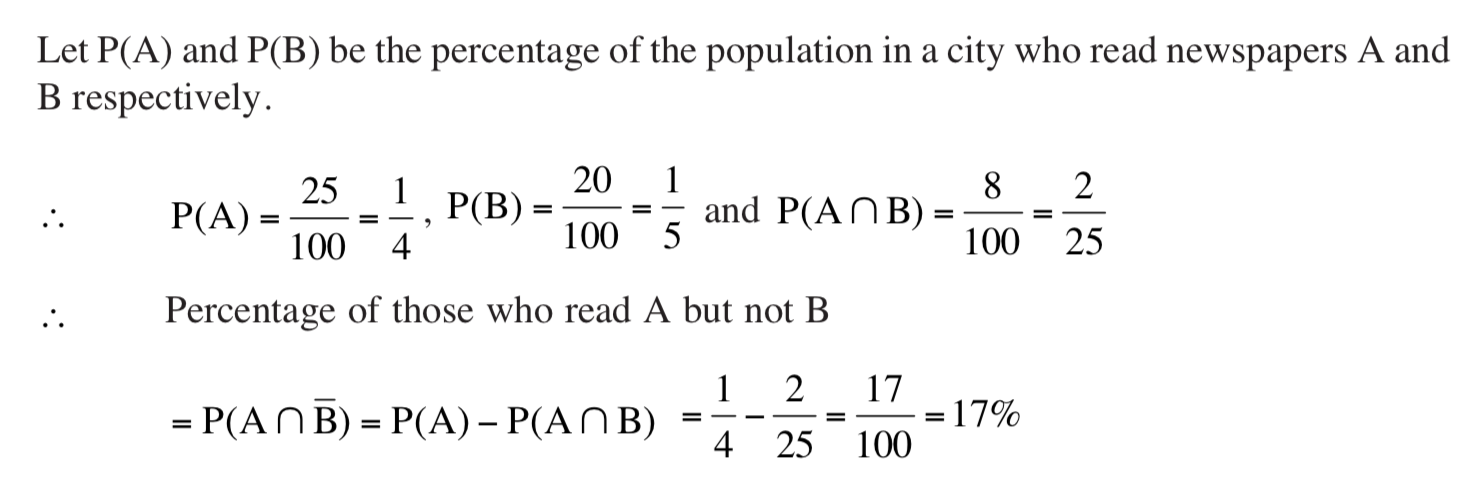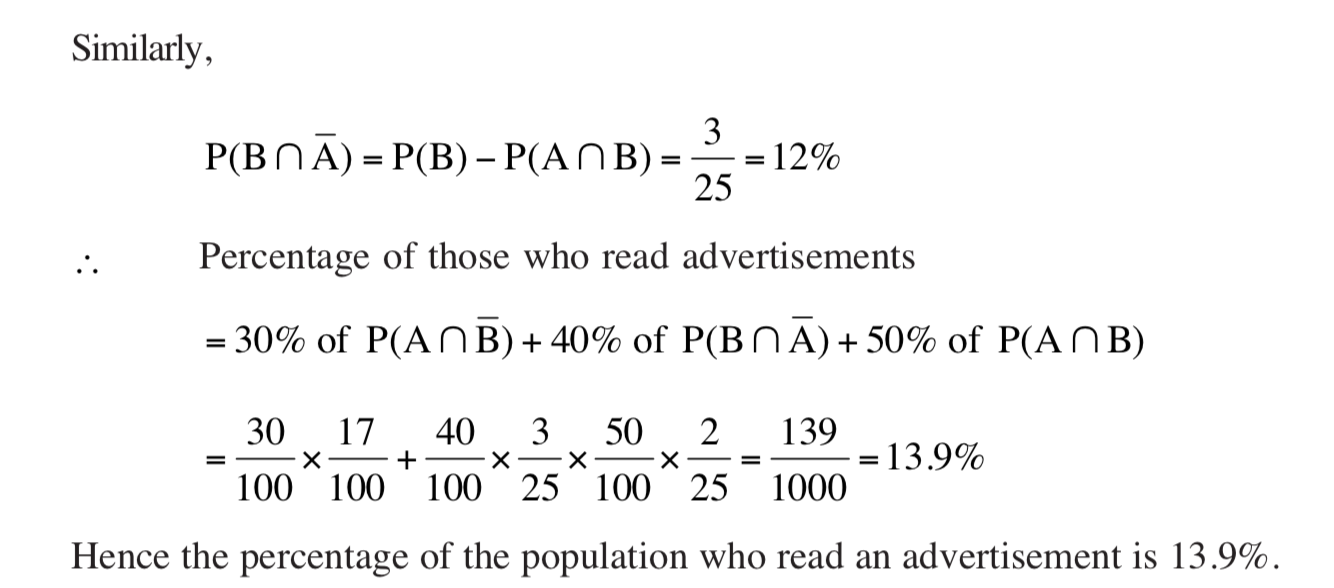# TOTAL PROBABILITY THEOREM

TOTAL PROBABILITY THEOREM

Let E1, E2, ..., En be n mutually exclusive and exhaustive events and event A is such that it can occur with any of the events E1, E2, E3, ....... En then the probability of the occurrence of event A can beExample: